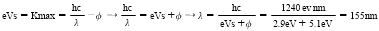# Untitled 1

Classified in Physics

Written at onEnglish with a size of 3.8 KB.

1) An experiment is setup to test the photoelectric effect with light incident on gold (the work function of gold is 5.1eV) The stopping potential is observed to be 2.9V. Find wavelength. Φ = 5.1eV, Vs=2.9v,2) A photon with an energy of 10Mev undergoes Compton Scattering. The recoiling electron has an energy of 7Mev. At what angle is the photon scattered?

3) Particle A decays to Particle B and a photon. The mass of Particle B is 91 GeV/c^2 and the velocity is 0.995c. What is the photon wavelength?

5) What is the value of the constant A such that the wave function is properly normalized?

2) A photon with an energy of 10Mev undergoes Compton Scattering. The recoiling electron has an energy of 7Mev. At what angle is the photon scattered?